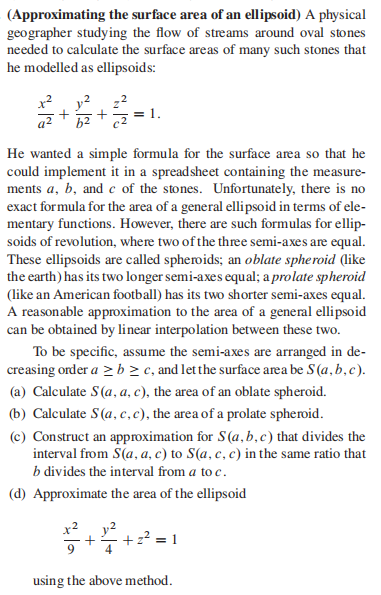### Still have math questions?

Algebra
Question(Approximating the surface area of an ellipsoid) A physical geographer studying the flow of streams around oval stones needed to calculate the surface areas of many such stones that he modelled as ellipsoids: $$\frac { x ^ { 2 } } { a ^ { 2 } } + \frac { y ^ { 2 } } { b ^ { 2 } } + \frac { z ^ { 2 } } { c ^ { 2 } } = 1$$ . He wanted a simple formula for the surface area so that he could implement it in a spreadsheet containing the measure- ments $$a , b$$ , and $$c$$ of the stones. Unfortunately, there is no exact formula for the area of a general ellipsoid in terms of ele- mentary functions. However, there are such formulas for ellip- soids of revolution, where two of the three semi-axes are equal. These ellipsoids are called spheroids; an oblate spheroid (like the earth) has its two longer semi-axes equal; a prolate spheroid (like an American football) has its two shorter semi-axes equal. A reasonable approximation to the area of a general ellipsoid can be obtained by linear interpolation between these two. To be specific, assume the semi-axes are arranged in de- creasing order $$a \geq b \geq c$$ , and let the surface area be $$S ( a , b , c$$ ).

(a) Calculate $$S ( a , a , c$$ ), the area of an oblate spheroid.

(b) Calculate $$S ( a , c , c$$ ), the area of a prolate spheroid.

(c) Construct an approximation for $$S ( a , b , c$$ ) that divides the interval from $$S ( a , a , c$$ ) to $$S ( a , c , c$$ in the same ratio that $$b$$ divides the interval from $$a$$ to $$c$$ .

(d) Approximate the area of the ellipsoid $$x ^ { 2 }$$

$$\left. \begin{array} { l } { \text { (a) } S ( a , a , c ) = 2 \pi a ^ { 2 } + \frac { 2 \pi a c ^ { 2 } } { \sqrt { a ^ { 2 } - c ^ { 2 } } } \ln ( \frac { a + \sqrt { a ^ { 2 } - c ^ { 2 } } } { c } ) } \\ { \text { (b) } S ( a , c , c ) = 2 \pi c ^ { 2 } + \frac { 2 \pi a ^ { 2 } c } { \sqrt { a ^ { 2 } - c ^ { 2 } } } \cos ^ { - 1 } ( \frac { c } { a } ) } \\ { \text { (c) } S ( a , b , c ) \approx \frac { b - c } { a - c } S ( a , a , c ) + \frac { a - b } { a - c } S ( a , c , c ) } \\ { \text { (d) } S ( 3,2,1 ) \approx 49.595 } \end{array} \right.$$